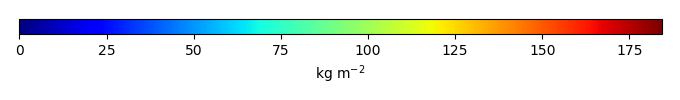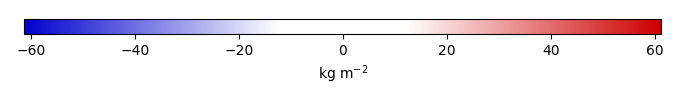# Mean State

Period Mean (original grids) [Pg]
Model Period Mean (intersection) [Pg]
Model Period Mean (complement) [Pg]
Benchmark Period Mean (intersection) [Pg]
Benchmark Period Mean (complement) [Pg]
Bias [kg m-2]
Bias Score 
Spatial Distribution Score 
Overall Score 
Benchmark [-] 76.5
CLM4 [-] 7.93 7.01 1.05 76.1 0.362 -24.3 0.41 0.20 0.30
CLM4.5 [-] 93.5 83.8 9.89 76.1 0.362 3.99 0.54 0.39 0.46
CLM5 [-] 248. 232. 16.7 76.1 0.362 57.1 0.24 0.075 0.16
Period Mean (original grids) [Pg]
Model Period Mean (intersection) [Pg]
Model Period Mean (complement) [Pg]
Benchmark Period Mean (intersection) [Pg]
Benchmark Period Mean (complement) [Pg]
Bias [kg m-2]
Bias Score 
Spatial Distribution Score 
Overall Score 
Benchmark [-] 113.
CLM4 [-] 85.6 45.5 38.1 113. 0.00185 -14.1 0.55 0.29 0.42
CLM4.5 [-] 156. 84.4 68.2 113. 0.00185 -6.10 0.62 0.83 0.73
CLM5 [-] 294. 194. 94.1 113. 0.00185 16.5 0.52 0.55 0.54
Period Mean (original grids) [Pg]
Model Period Mean (intersection) [Pg]
Model Period Mean (complement) [Pg]
Benchmark Period Mean (intersection) [Pg]
Benchmark Period Mean (complement) [Pg]
Bias [kg m-2]
Bias Score 
Spatial Distribution Score 
Overall Score 
Benchmark [-] 12.2
CLM4 [-] 86.3 3.55 84.7 12.2 0.00 -8.82 0.50 0.78 0.64
CLM4.5 [-] 118. 6.54 115. 12.2 0.00 -5.53 0.60 0.85 0.73
CLM5 [-] 122. 13.3 113. 12.2 0.00 1.52 0.53 0.26 0.40
Period Mean (original grids) [Pg]
Model Period Mean (intersection) [Pg]
Model Period Mean (complement) [Pg]
Benchmark Period Mean (intersection) [Pg]
Benchmark Period Mean (complement) [Pg]
Bias [kg m-2]
Bias Score 
Spatial Distribution Score 
Overall Score 
Benchmark [-] 97.3
CLM4 [-] 5.53 5.24 0.729 97.2 0.0996 -29.1 0.39 0.11 0.25
CLM4.5 [-] 95.5 87.9 8.96 97.2 0.0996 -2.82 0.62 0.63 0.62
CLM5 [-] 254. 242. 14.9 97.2 0.0996 46.5 0.41 0.10 0.26
Period Mean (original grids) [Pg]
Model Period Mean (intersection) [Pg]
Model Period Mean (complement) [Pg]
Benchmark Period Mean (intersection) [Pg]
Benchmark Period Mean (complement) [Pg]
Bias [kg m-2]
Bias Score 
Spatial Distribution Score 
Overall Score 
Benchmark [-] 5.12
CLM4 [-] 24.8 0.594 23.8 5.12 0.00 -16.8 0.41 0.076 0.24
CLM4.5 [-] 57.3 2.92 53.6 5.12 0.00 -8.21 0.59 0.44 0.52
CLM5 [-] 67.9 4.90 62.0 5.12 0.00 -0.930 0.70 0.89 0.80
Period Mean (original grids) [Pg]
Model Period Mean (intersection) [Pg]
Model Period Mean (complement) [Pg]
Benchmark Period Mean (intersection) [Pg]
Benchmark Period Mean (complement) [Pg]
Bias [kg m-2]
Bias Score 
Spatial Distribution Score 
Overall Score 
Benchmark [-] 475.
CLM4 [-] 614. 99.9 514. 474. 1.51 -16.4 0.46 0.21 0.34
CLM4.5 [-] 1.27e+03 418. 853. 474. 1.51 -2.02 0.57 0.77 0.67
CLM5 [-] 1.92e+03 1.08e+03 836. 474. 1.51 27.6 0.43 0.19 0.31
Period Mean (original grids) [Pg]
Model Period Mean (intersection) [Pg]
Model Period Mean (complement) [Pg]
Benchmark Period Mean (intersection) [Pg]
Benchmark Period Mean (complement) [Pg]
Bias [kg m-2]
Bias Score 
Spatial Distribution Score 
Overall Score 
Benchmark [-] 116.
CLM4 [-] 23.0 21.4 1.40 116. 0.373 -15.4 0.44 0.15 0.29
CLM4.5 [-] 126. 117. 8.33 116. 0.373 1.37 0.53 0.72 0.62
CLM5 [-] 326. 313. 14.0 116. 0.373 34.5 0.41 0.15 0.28
Period Mean (original grids) [Pg]
Model Period Mean (intersection) [Pg]
Model Period Mean (complement) [Pg]
Benchmark Period Mean (intersection) [Pg]
Benchmark Period Mean (complement) [Pg]
Bias [kg m-2]
Bias Score 
Spatial Distribution Score 
Overall Score 
Benchmark [-] 0.0131
CLM4 [-] 33.2 0.0213 32.7 0.0131 0.00 3.77 0.53 4.0e-23 0.26
CLM4.5 [-] 53.3 0.0389 52.5 0.0131 0.00 11.6 0.14 6.2e-23 0.070
CLM5 [-] 42.8 0.0285 42.1 0.0131 0.00 6.98 0.31 3.3e-20 0.15
Period Mean (original grids) [Pg]
Model Period Mean (intersection) [Pg]
Model Period Mean (complement) [Pg]
Benchmark Period Mean (intersection) [Pg]
Benchmark Period Mean (complement) [Pg]
Bias [kg m-2]
Bias Score 
Spatial Distribution Score 
Overall Score 
Benchmark [-] 46.1
CLM4 [-] 41.7 16.2 25.7 46.1 0.00725 -14.4 0.51 0.13 0.32
CLM4.5 [-] 75.3 32.9 42.8 46.1 0.00725 -6.41 0.56 0.33 0.45
CLM5 [-] 145. 81.7 61.9 46.1 0.00725 16.6 0.54 0.68 0.61
Period Mean (original grids) [Pg]
Model Period Mean (intersection) [Pg]
Model Period Mean (complement) [Pg]
Benchmark Period Mean (intersection) [Pg]
Benchmark Period Mean (complement) [Pg]
Bias [kg m-2]
Bias Score 
Spatial Distribution Score 
Overall Score 
Benchmark [-] 0.232
CLM4 [-] 11.8 0.309 11.9 0.232 0.00 1.08 0.49 0.45 0.47
CLM4.5 [-] 14.5 0.310 14.9 0.232 0.00 1.08 0.49 0.72 0.61
CLM5 [-] 12.9 0.398 13.2 0.232 0.00 1.94 0.60 0.71 0.65

# Temporally integrated period mean

BENCHMARK MEANMODEL MEANBIASBIAS SCORESPATIAL TAYLOR DIAGRAMMODEL COLORS# Spatially integrated regional mean

MODEL COLORS# All Models

BenchmarkCLM4CLM4.5CLM5# Data Information

Approach: I just read the data from the original data file and converted the latitude arrange from original 90N->90S to 90S->90N. The unit was convert from original 0.1 KgC/m2 to KgC/m2

Temporal resolution: yearly

General information: This product was derived from Northern Circumpolar Soil Carbon Database V2.2 (NCSCDv22) in top 1m.

Spatial resolution: 0.5x0.5 degree